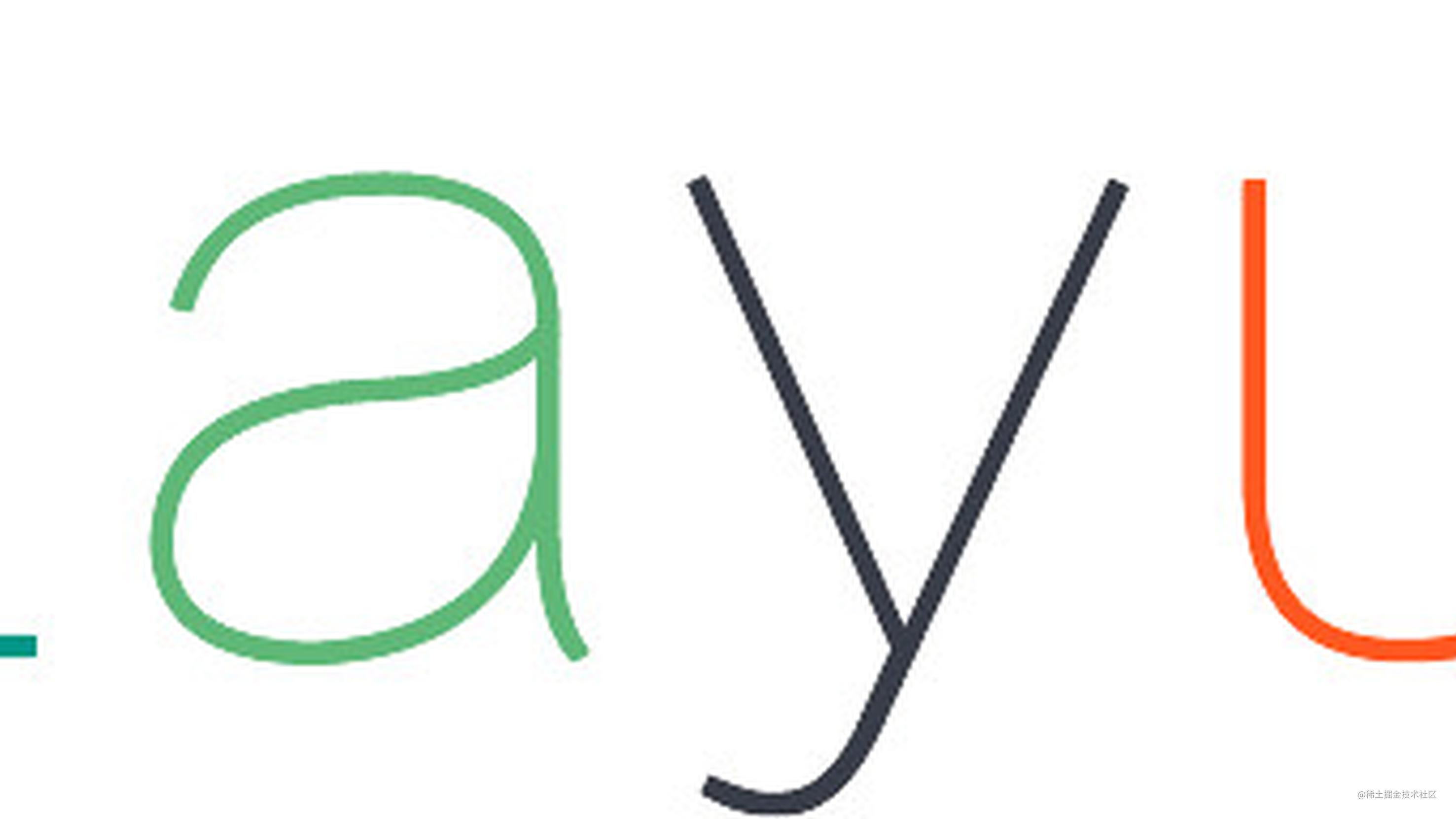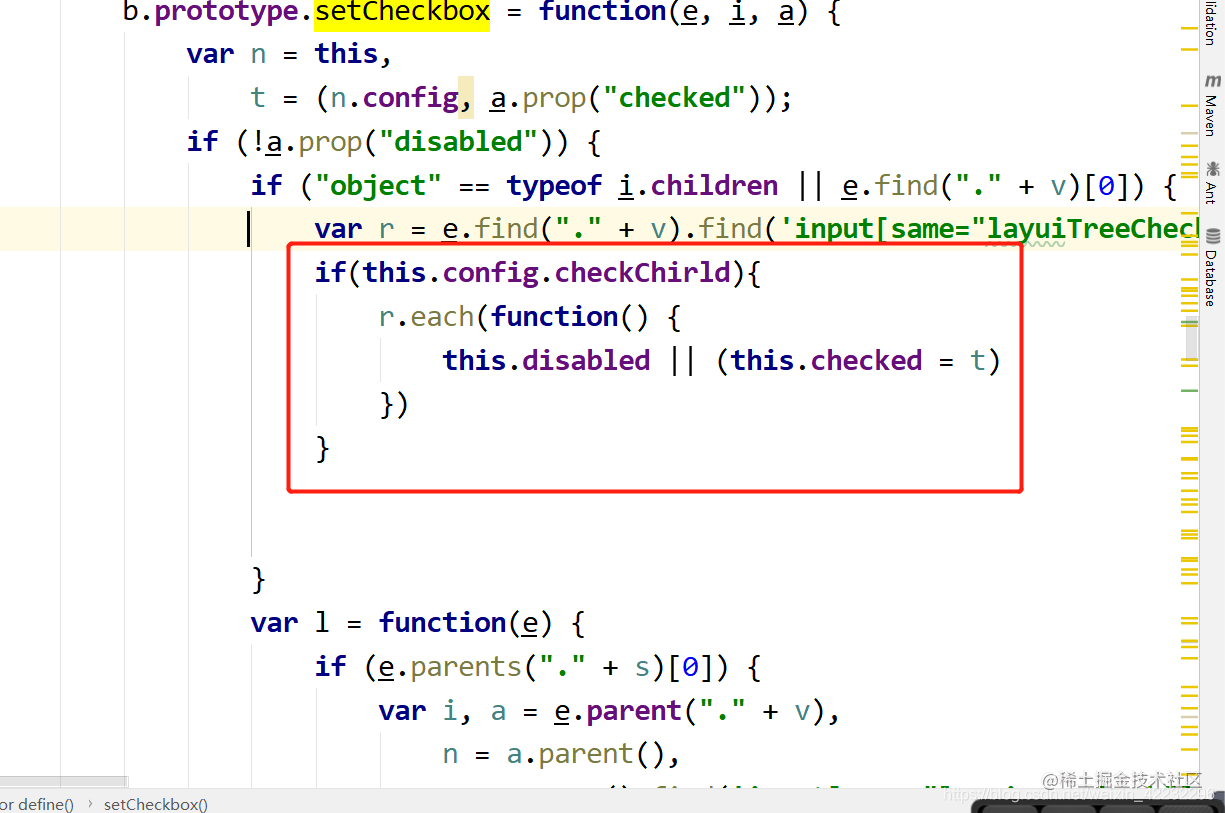# layui tree组件 回显bug(只要勾选一个子集在回显的时候会勾选全部子集)## 解决思路

1.打破这种关联，让选中父级元素的时候可以控制是否关联子集元素。 在tree.js中全局搜索以下代码（压缩过的子集格式化下代码），加上如下判断

``````b.prototype.setCheckbox = function(e, i, a)实例化tree的时候，多添加一个配置项checkChirld

``````  tree.render({
elem: '#permissionTree'
,checkChirld:false //是否关联子集菜单
,data: [[\${tree}]]
,showCheckbox: true  //是否显示复选框
,id: 'treeId'
,isJump: false //是否允许点击节点时弹出新窗口跳转
,showLine: false
});

`````` b.prototype.render = function() {
var e = this,
a = e.config;
e.checkids = [];
var n = i('<div class="layui-tree' + (a.showCheckbox ? " layui-form": "") + (a.showLine ? " layui-tree-line": "") + '" lay-filter="LAY-tree-' + e.index + '"></div>');
e.tree(n);
var t = a.elem = i(a.elem);
if (t) {
if (e.key = a.id || e.index, e.elem = n, e.elemNone = i('<div class="layui-tree-emptyText">' + a.text.none + "</div>"), t.html(e.elem), 0 == e.elem.find(".layui-tree-set").length) return e.elem.append(e.elemNone);
a.showCheckbox && e.renderForm("checkbox"),
e.elem.find(".layui-tree-set").each(function() {
var e = i(this);
}),
e.events()
}
this.config.checkChirld = true;
},